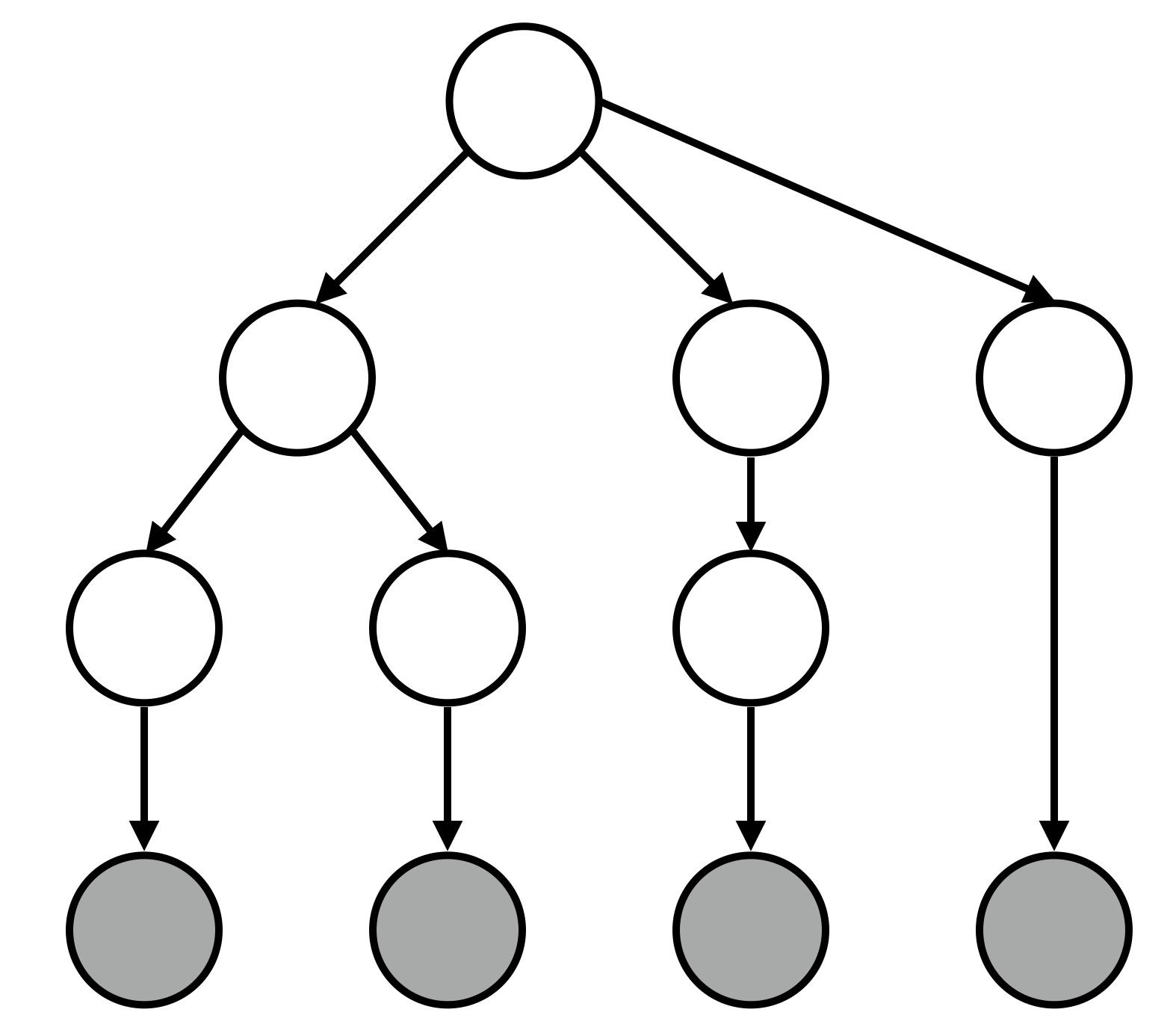Whereas HMMs assume that sequence elements are generated from hidden variables organized in a flat linear structure, PCFGs assume that the hidden variables can be organized hierarchically. This allows for richer representations. For instance, an HMM would have a hard time with the sentence:

Joan saw the big old scary man with binoculars.

Here, there are two possible readings (either Joan has the binoculars or the man does) but an HMM would have a hard time capturing this because the information involved in this inference is so far apart; “Joan” is at the beginning of the sentence and “with binoculars” is at the end.

# Sampling from a PCFG

``````// extract words from a single string
// assumes that whitespace is the delimiter
var w = function(string) {
return string.split(/ +/);
}

var grammar = {
"S":  map(w, ["x OP S", "S OP x", "N"]),
"OP": map(w, ["+", "-", "*"]),
"N":  map(w, ["0", "1", "2", "3", "4", "5", "6"])
};

var isTerminal = function(symbol) {
return !(_.contains(["S","OP","N"], symbol))
}

var unfold = function(symbol) {
if (isTerminal(symbol)) {
return symbol
}

var rules = grammar[symbol];
var sampledRule = rules[ randomInteger(rules.length) ];

return [symbol].concat( map(unfold, sampledRule) );
}

var reformatTree = function(x) {
if (Array.isArray(x)) {
return "[" + map(reformatTree, x).join(" ") + "]"
}
return x
}

repeat(5,
function() { return reformatTree(unfold("S")) })
``````

(Plug in the output of reformatTree to Miles Shang’s syntax tree generator for visualization)

# Application: parsing ambiguous sentences

``````/*

S -> NP VP
NP -> N | D N | D N PP
VP -> V NP | V' PP
V' -> V NP
PP -> P NP

*/

var w = function(string) {
return string.split(/ +/);
}

var grammar = {
"S":  map(w, ["NP VP"]),
"NP": map(w, ["N", "D N", "D N PP"]),
"VP": map(w, ["V NP", "Vb PP"]),
"Vb": map(w, ["V NP"]),
"PP": map(w, ["P N"]),
"P":  map(w, ["with"]),
"N":  map(w, ["Joan", "man", "binoculars"]),
"V":  map(w, ["saw"]),
"D":  map(w, ["the"])
};

// get the leaves of a parse tree
var getLeaves = function(x) {
if (x.length > 1) {
return reduce(function(x, acc) { return getLeaves(x).concat(acc)},
[],
x.slice(1));
}

return [ x ]
}

var reformatTree = function(x) {
if (Array.isArray(x)) {
return "[" + map(reformatTree, x).join(" ") + "]"
}
return x
}

var preTerminals = ["P", "N", "V", "D"];
var terminals = _.flatten(map(function(x) { return grammar[x]},
["P", "N", "V", "D"]
));

// is a symbol a terminal?
var isTerminal = function(symbol) {
return _.contains(terminals, symbol);
}

// is a symbol a pre-terminal?
// i.e., does it only rewrite to terminals?
var isPreTerminal = function(symbol) {
return _.contains(["P","N","V","D"], symbol);
}

// rewrite a preterminal to a terminal
var samplePreTerminal = function(symbol) {
var rules = grammar[symbol];
return rules[ randomInteger(rules.length) ];
}

var _unfold = function(symbol) {
var yieldLeft = globalStore.yieldLeft;
if (yieldLeft.length == 0) {
factor(-Infinity);
}
if (isPreTerminal(symbol)) {
var terminal = samplePreTerminal(symbol);
factor(terminal == yieldLeft ? 0 : -Infinity);
globalStore.yieldLeft =  yieldLeft.slice(1);
return [symbol, terminal];
}
var rules = grammar[symbol];
var sampledRewrite = rules[ randomInteger(rules.length) ];
return [symbol].concat(map(_unfold, sampledRewrite));

};

var unfold = function() {
return _.flatten(_unfold("S")).join(" ");
}

var erp = ParticleFilter(function() {
globalStore.yieldLeft = "Joan saw the man with binoculars".split(" ");
var x = _unfold("S");
factor(globalStore.yieldLeft.length == 0 ? 0 : -Infinity);
return x;
}, 1000);

reformatTree( sample(erp) )
``````

(Plug in the output of reformatTree to Miles Shang’s syntax tree generator for visualization)